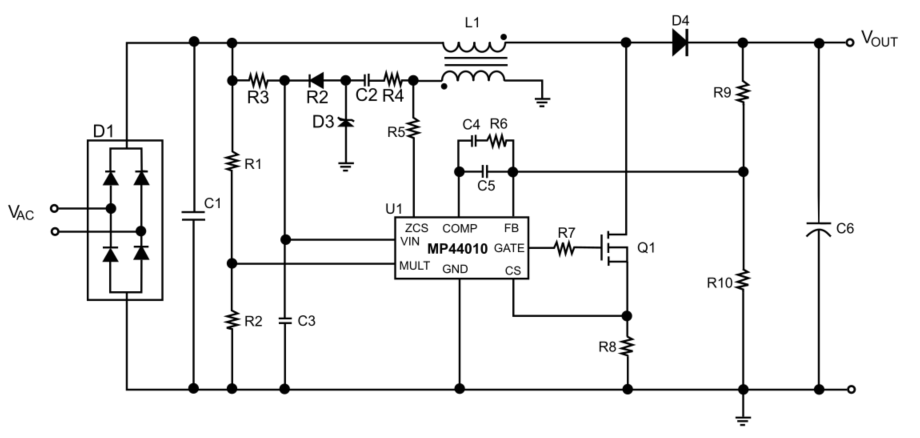# 功率因数校正(PFC)• 位移：当电路的电压和电流波形异相时会产生位移，通常是由电感或电容等电抗元件引起的。
• 失真：波的原始形状发生改变，通常是由整流器等非线性电路引起的。这些非线性波包含很多谐波含量，会使电网中的电压失真。

• 无源功率因数校正（PFC）：使用无源滤波器滤除谐波以提高功率因数。这种方法适用于低功率应用，在高功率应用中，其效果远远不够。
• 有源功率因数校正（PFC）：使用开关变换器调制失真波，以将其整形为正弦波。整形后的信号中存在的唯一谐波位于开关频率处，因此很容易滤除。有源功率因数校正被认为是最好的功率因数校正方法，但会增加设计的复杂性。

## AC / DC电源中的功率因数校正（PFC）需求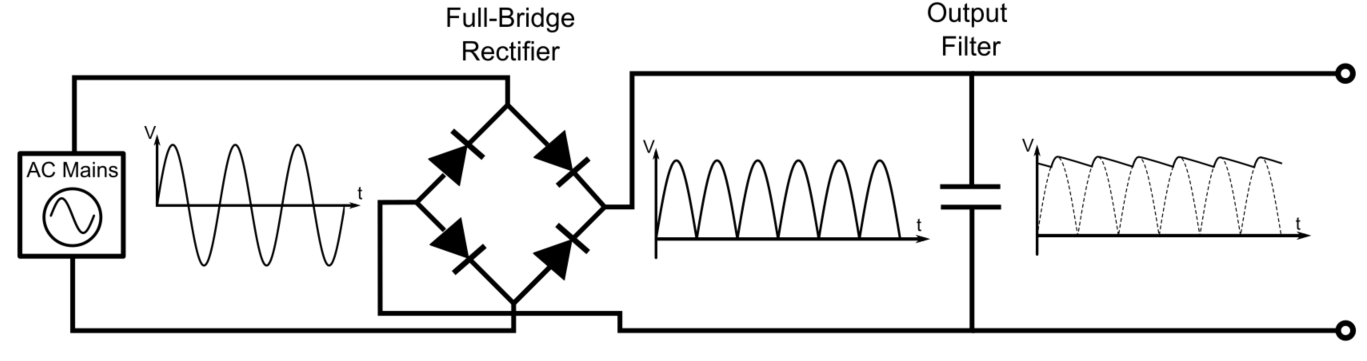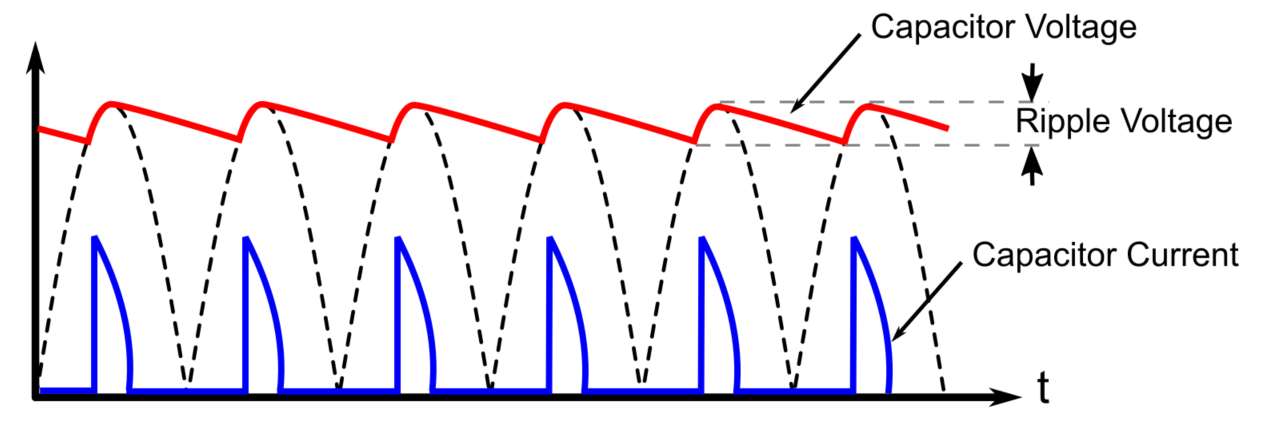## 谐波与傅立叶变换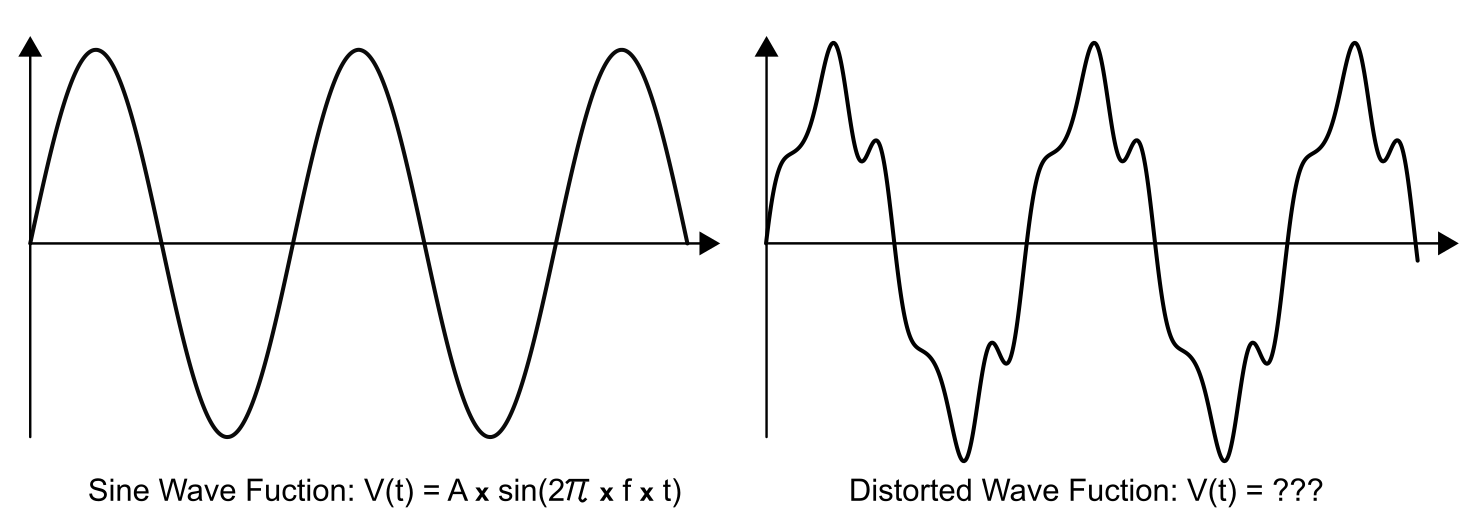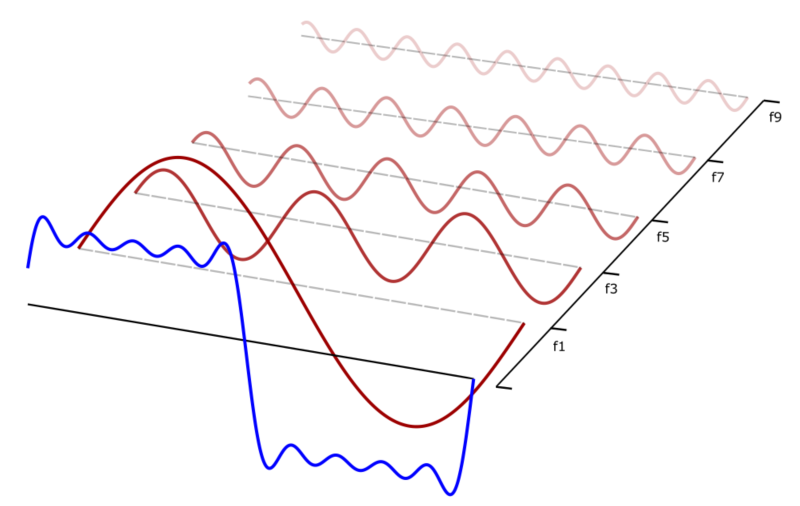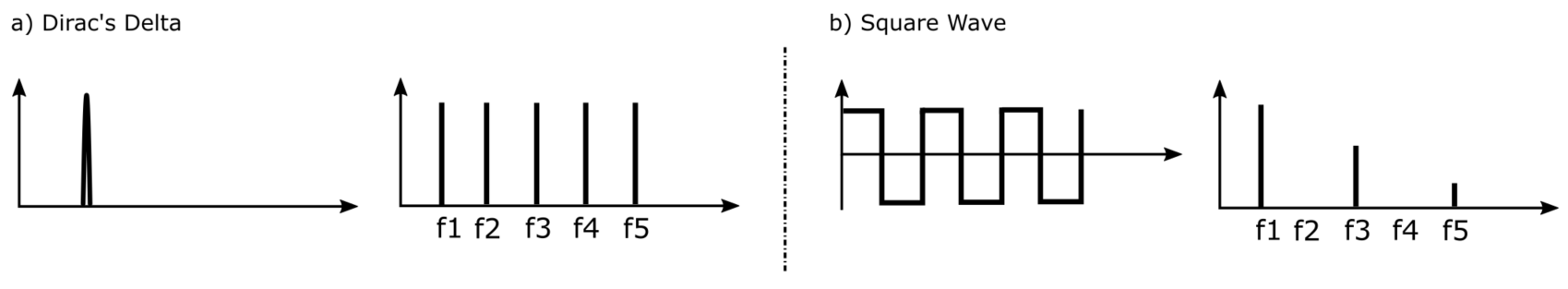## 功率因数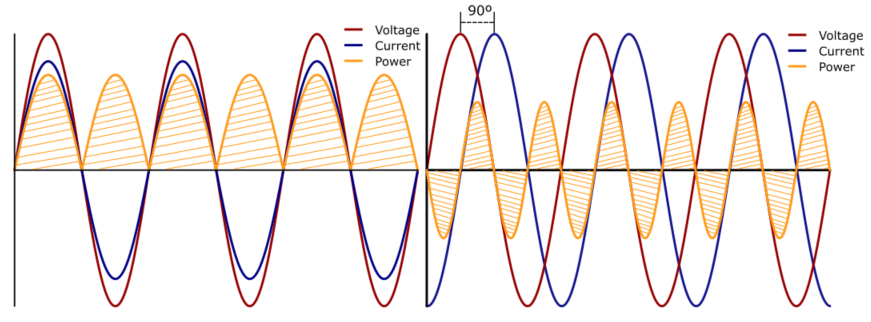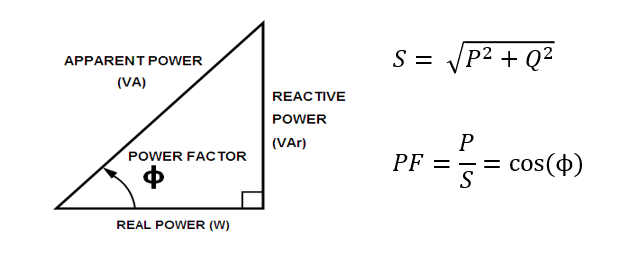$$PF_{DISPLACEMENT}= COS (\theta_{V}-\theta_{I})$$

$$THD = \frac {\sqrt {\sum n ≠1 \space I_{n}^2 \space RMS}}{ {I_{fund. \space RMS}}} = \frac {RMS \space harmonic \space current}{RMS \space fundamental \space current}$$

$$PF_{DISTORTION}= \sqrt \frac {1}{1+THD^2}$$

$$PF = PF_{DISPLACEMENT}\space x \space PF_{DISTORTION}= COS(\theta_{V}-\theta_{I}) \space x \space \sqrt \frac {1}{1+THD^2}$$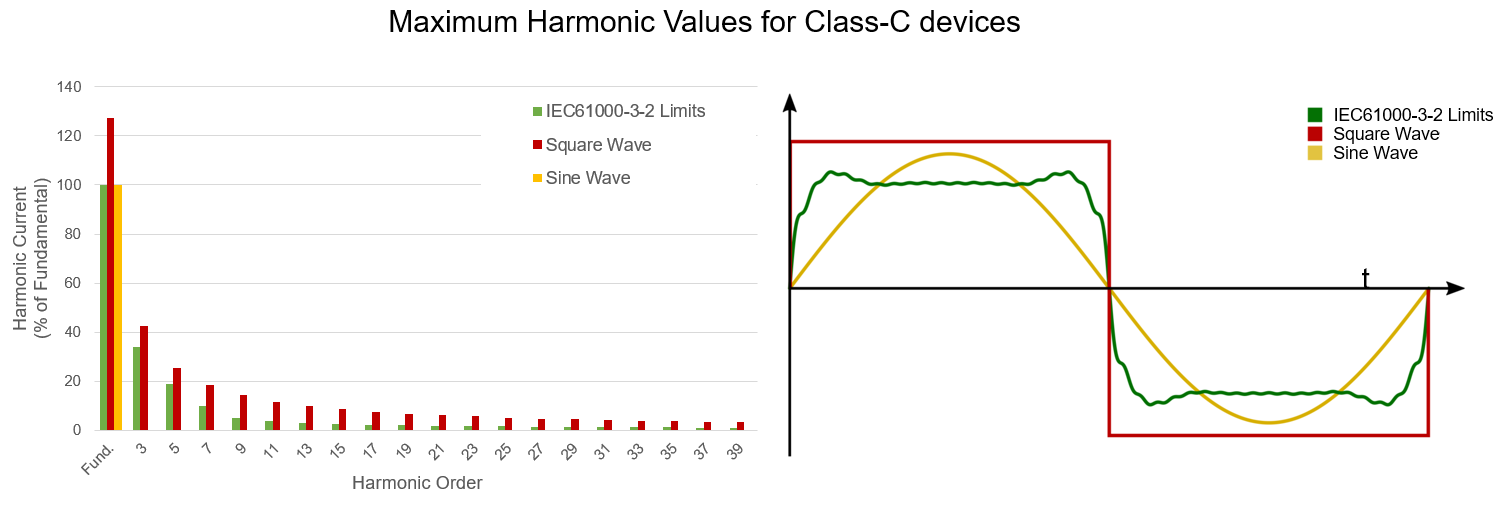## 什么是功率因数校正（PFC）?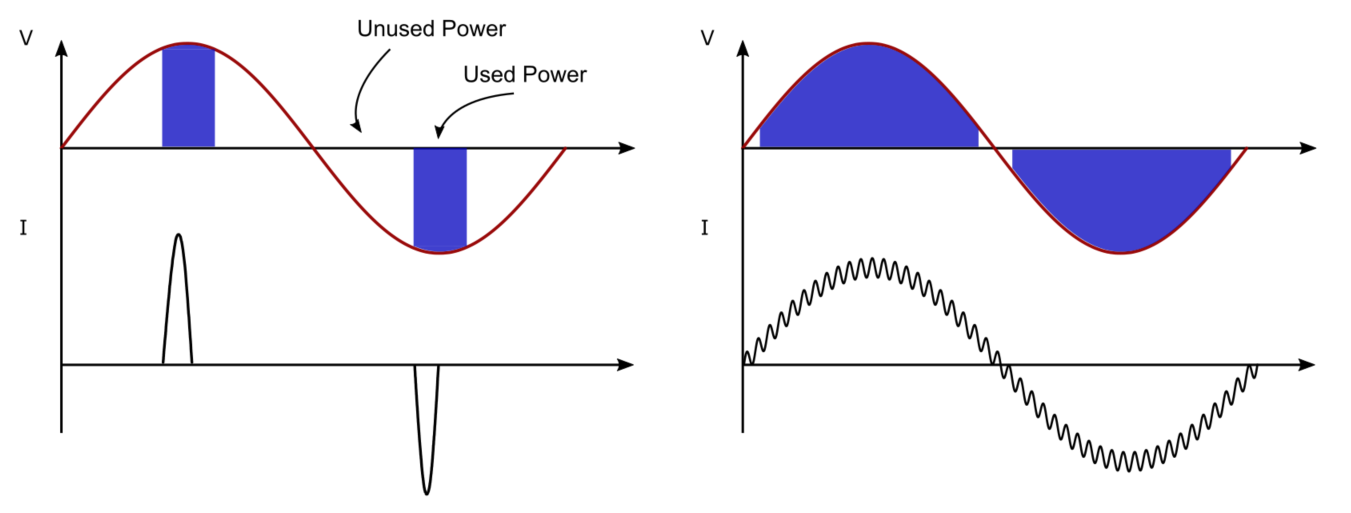1. 2. 滤除谐波：接受效率的损失，但尝试通过在输入处添加滤波器来减少注入电网的谐波数量。这称为无源PFC，它使用低通滤波器来滤除高次谐波，理想情况下仅保留50Hz基波（见图10）。在实际应用中，这种方法改善设备功率因数的效率较低，也不适用于大功率方案，因为添加的电容和电感会造成效率、尺寸和重量方面的损耗。它通常不会用于功率超过数百瓦的应用。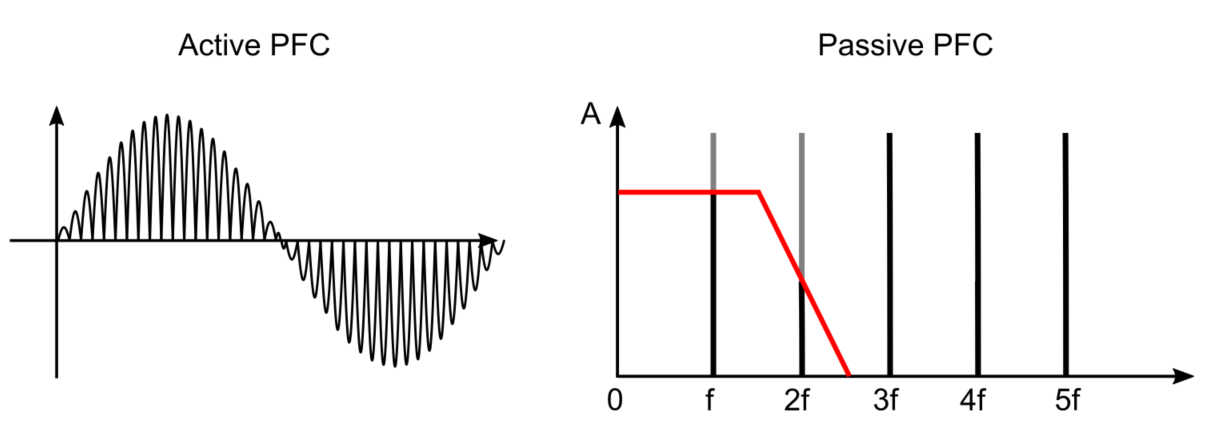1. 2. 有源功率因数校正。这种方法可以改变电流波形的形状，使其跟随电压。 这样，谐波被移到更高的频率上，因而更容易被滤除。使用最广泛的有源功率因数校正电路是升压变换器（见图11）。它与变压器类似，可以升高直流电压，同时降低电流。最简单的升压变换器由电感、晶体管和二极管组成。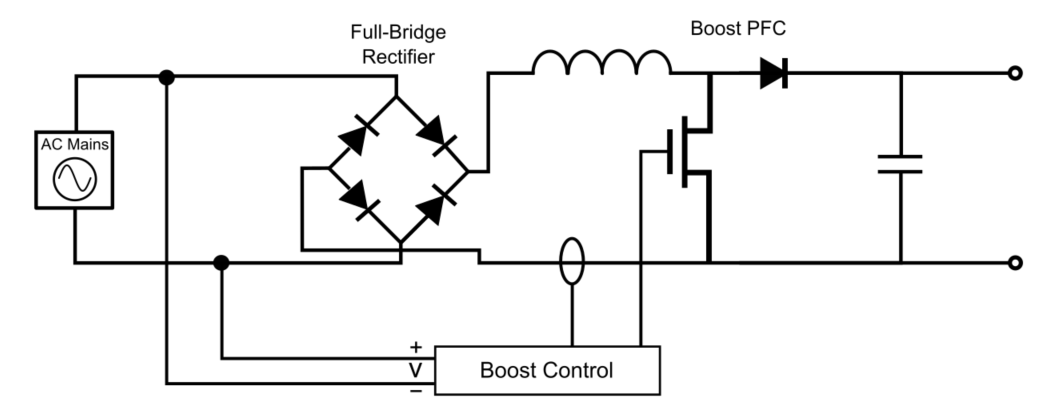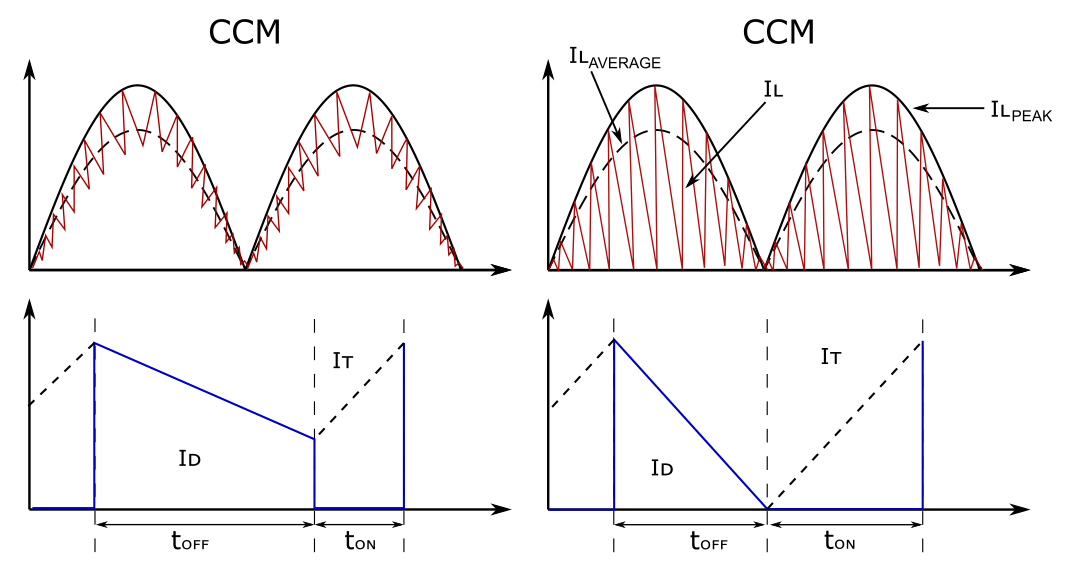MPS提供的MP44010控制器即为一款BCM功率因数校正器。当连接到升压变换器时，其ZCS引脚检测电感何时放电并激活MOSFET（如图13中的Q1）。该器件还可以比较电流和电压，调整电流峰值以跟随输入电压的波形。